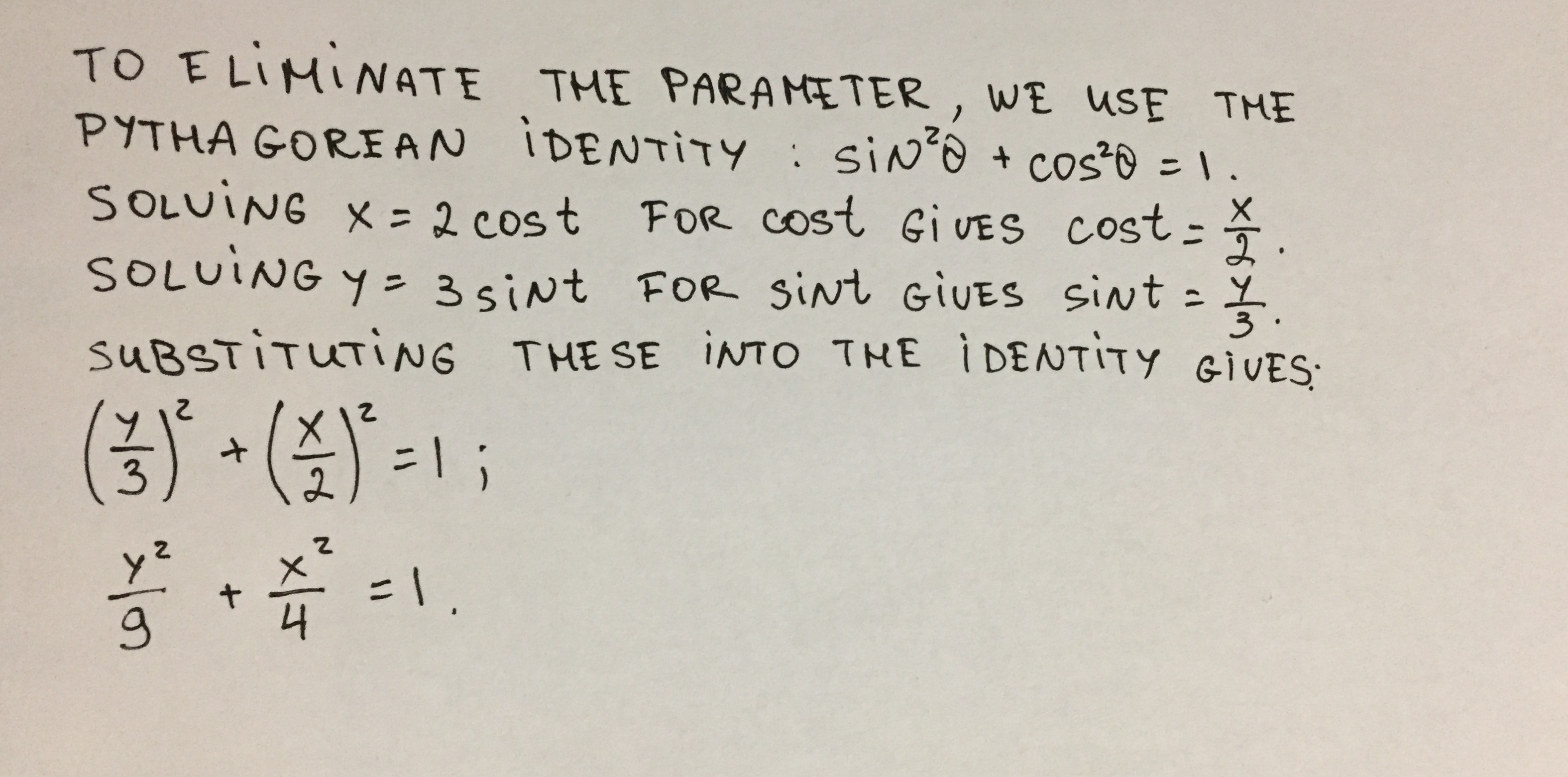Question# A pair of parametric equations is given. Find a rectangular-coordinate

Equation, expression, and inequalitie
ANSWEREDA pair of parametric equations is given. Find a rectangular-coordinate equation for the curve by eliminating the parameter.
$$\displaystyle{x}={2}{\cos{{t}}},{y}={3}{\sin{{t}}},{0}≤{t}≤{2}π$$2021-08-15

To eliminate the parameter, we use the Pythagorean Identity $$\sin^2 θ + \cos^2 θ = 1$$. Solving $$x = 2\cos t$$ for $$\cos t$$ gives $$\cos t = \frac{x}{2}$$. Solving $$y = 3\sin t$$ for $$\sin t$$ gives $$\sin t = \frac{y}{3}$$. Substituting these into the identity then gives:

$$(\frac{y}{3})^2 + (\frac{x}{2})^2 = 1$$

$$\frac{y^2}{9} + \frac{x^2}{4} = 1$$